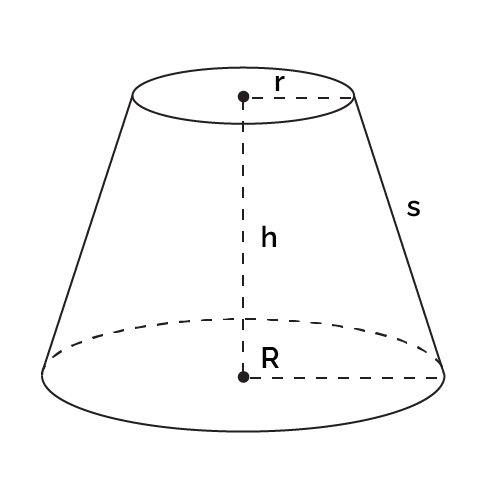# Truncated Cone Calculator

Created by Anna Szczepanek, PhD
Reviewed by Komal Rafay
Last updated: Aug 22, 2022

This truncated cone calculator is a comprehensive tool for solving various problems related to truncated cones. Our tool will teach you how to find the surface area of a truncated cone: both the lateral area and the total area. Of course, it can compute the slant height and the volume as well. Its best feature though is that it can work in reverse: input whatever data you have and get the missing measurements. No more crying over truncated cones in geometry class. Enjoy!

## What is a truncated cone in geometry?

A truncated cone is a cone with the apex cut off. Importantly, the cutting plane is parallel to the base, or, equivalently, perpendicular to the height. This solid is also known under the name frustum. In everyday life, you can see truncated cones looking at, e.g., lamp shades.

Now, let's move on to discussing truncated cone formulas.

## Truncated cone formulas

First, we need to establish some notation. Look at the picture below. We have:

• R for the radius of the base (larger circle);
• r for the radius of the top surface (smaller circle);
• h for the height, i.e., the shortest perpendicular distance between the two circles; and
• s for the slant height, i.e., the distance between the two circles measured along the lateral face.Volume formula:

V = (1/3) × π × h × (r² + r × R + R²).

Slant height formula:

s = √((R - r)² + h²).

Lateral surface area formula:

Lateral = π × (R + r) × s.

Top and bottom surface area:

Top = π × r² and Bottom = π × R².

Total area formula:

Total = Lateral + Top + Bottom.

Wow, that's a lot of formulas. But hey, you don't need to learn them by heart! We've implemented them in our truncated cone calculator to save your time and effort.

## How to use this truncated cone calculator?

To use this truncated cone calculator, you only need to enter the data: the remaining fields will get calculated immediately. Note that you can adjust the units to your needs!

Did you get a red warning? This means the data you entered is contradictory - please check it carefully and correct any mistakes.

Interested in cones and similar stuff? Here's a few Omni tools where you can discover more:

## FAQ

### What is the slant height of a truncated cone with height 4 and radii 1 and 4?

The answer is 5. The slant height formula reads:

s = √((R - r)² + h²).

Plugging in our data, we get:

s = √((4 - 1)² + 4²) = √25 = 5.

### How do I get the slant height formula for a truncated cone?

Use the Pythagorean theorem to derive the slant height formula. The slant height is the hypotenuse. One of the legs is the difference between the longer and the shorter radius of the truncated cone. The other leg is the height of the truncated cone.

### How do I calculate the lateral surface area of a truncated cone with slant height 4?

You need to know the radii to find the area. If the radii are 10 and 12, then the lateral surface area is 276.46. To derive this answer:

1. Add together the radii: 10 + 12 = 22.
2. Multiply the result by the slant height: 22 × 4 = 88.
3. Multiply by π: 88π ≈ 276.46.
4. Remember to include the units: if the dimensions are in cm, then the answer is 276.46 cm².
Anna Szczepanek, PhDHeight (h)
in
in
in
Slant height (s)
in
Volume
cu in
Lateral surface area
in²
Top surface area
in²
Bottom surface area
in²
Total surface area
in²
People also viewed…

### Long division

Check this long division calculator if you want to solve any division problem and see all the steps towards the result.

### Mixed number to improper fraction

This mixed number to improper fraction calculator works like a charm - it not only converts one fraction form to the other, but also it shows the step by step solution.

### Social Media Time Alternatives

Check what you could have accomplished if you get out of your social media bubble.

### Sunbathing

Do you always remember to put on sunscreen before going outside? Are you sure that you use enough? The Sunbathing Calculator ☀ will tell you when's the time to go back under an umbrella not to suffer from a sunburn!